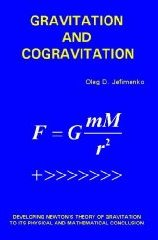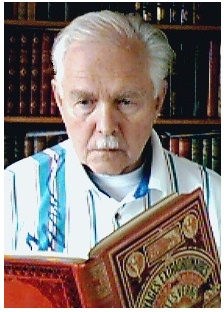Enter the content which will be displayed in sticky bar

# BookPages: 384
Publisher: Electret Scientific Company
Year: 2006
ISBN: 091740615X
ISBN: 978-0917406157

Gravitation and Cogravitation: Developing Newton's Theory of Gravitation to its Physical and Mathematical Conclusion (Buy Now)
KeyWords: gravity
Authors
•Professor Emeritus of Physics
(Relativity, Electromagnetic Retardation, Gravity)

Born in Kharkov, Ukraine, Oleg D. Jefimenko came to the US in 1951.  Education: Vordiplom, U. Goettingen, Germany, 1950; BA, Lewis and Clark Coll., 1952; MA, U. Oreg., 1954; PhD, U. Oreg., 1956. He spent his entire career since 1956 as Assisitant, Associate, and ultimately Professor of Physics in 1966 at the University of West Virginia in Morgantown. His 1966 textbook, Electricity and Magnetism, famous for its Jefimenko equations, has remained in print through several editions.

I am currently engaged in the development of the theory of electromagnetic retardation and relativity. Perhaps the most important recently discovered aspect of the now evolving theory of electromagnetic retardation is that this theory leads to, and duplicates, many electromagnetic relations that are customarily considered to constitute consequences of relativistic electrodynamics. It is now clear that relativistic electromagnetism and relativistic mechanics can be derived from Maxwellian electromagnetic theory and electromagnetic force relations via the theory of electromagnetic retardation without any postulates, conjectures, or hypotheses. As a result, the theory of relativity acquires a new mathematical and physical base which provides a previously unforeseen means for formulating, developing, and interpreting relativistic electromagnetism, relativistic mechanics, and relativistic gravitation.

I am also working on the generalization of Newton\'s gravitational theory to time-dependent systems. By analyzing causal gravitational relations, I find that there is no objective reason for abandoning Newton\'s force-field gravitational theory in favor of a metric gravitational theory, and that Newton\'s theory must be developed and expanded to its logical and mathematical conclusion by making it compatible with the principle of causality and by making it applicable to time-dependent gravitational interactions. I base such an expansion, or generalization, on the existence of the second gravitational force field, the \"cogravitational, or Heaviside\'s, field\" (except for a numerical factor, the cogravitational field is the same as the \"gravimagnetic\" field of the general relativity theory). This field was first predicted by Oliver Heaviside in his 1893 article \"A Gravitational and Electromagnetic Analogy\" (click HEAVISIDE to see this remarkable but, regrettably, long-neglected article).

The fundamental equations of the generalized Newton\'s theory are causal gravitational equations expressing time-dependent gravitational and cogravitational fields in the form of retarded integrals. For time- independent systems, these equations reduce to the conventional equations of the original Newton\'s theory. An important aspect of the generalized Newton\'s theory is that gravitational interactions involve not one single force of attraction but at least five different forces. Several consequences of the generalized Newton\'s theory are similar to certain consequences of Einstein\'s general relativity theory. An important consequence of the generalized Newton\'s theory is the possibility of the existence of stellar antigravitational mass configurations.

Articles (Selected):

1. \"Force Exerted on a Stationary Charge by a Moving Electric Current or by a Moving Magnet,\" American Journal of Physics, V61, p. 218-222 (1993).
2. \"Torque exerted by a moving electric charge distribution on a stationary electric charge distribution,\" J. Phys. A: Math. Gen. 32, 5305- 5314 (2002).
3. \"Dynamic electric field maps,\" The Physics Teacher, 38, 154-157 (2000).
4. \"On the relativistic invariance of Maxwell\'s equations,\" Z. Naturforsch. 54a 637-644 (1999).
5. \"The Trouton-Noble paradox,\" J. Phys. A: Math. Gen. 32, 3755- 3762 (1999).
6. \"A relativistic paradox seemingly violating conservation of momentum law in electromagnetic systems,\" Eur. J. Phys. 20, 39-44 (1999).
7. \"On the experimental proofs of relativistic length contraction and time dilation,\" Z. Naturforsch. 53a, 977-982 (1998).
8. \"On Maxwell\'s displacement current,\" Eur. J. Phys. 19, 469-470 (1998).
9. \"Correct use of Lorentz-Einstein transformation equations for electromagnetic fields,\" Eur. J. Phys. 18, 444-447 (1997).
10. \"Is magnetic field due to an electric current a relativistic effect?\" Eur. J. Phys. 17, 180-182 (1996).
11. \"Retardation and relativity: new integrals for electric and magnetic potentials of time-independent charge distributions moving with constant velocity,\" Eur. J. Phys. 17, 258-264 (1996).
12. \"Direct calculation of time dilation,\" Am. J. Phys. 64, 812-814 (1996).
13. \"Derivation of relativistic force transformation equations from Lorentz force law,\" Am J. Phys. 64, 618-620 (1996).
14. \"The nature of electromagnetic induction,\" Galilean Electrodynamics 6, 83-86 (1995).
15. \"Retardation and relativity: Derivation of Lorentz-Einstein transformations from retarded integrals for electric and magnetic fields,\" Am. J. Phys. 63, 267-272 (1995).
16. \"Retardation and relativity: The case of a moving line charge,\" Am. J. Phys. 63, 454-459 (1995).
17. \"Derivation of relativistic transformations for gravitational fields from retarded integrals,\" Galilean Electrodynamics 6, 23-30 (1995).
18. \"Gravitational field of a point mass moving with uniform linear or circular velocity,\" Galilean Electrodynamics 5, 25-33 (1994).
19. \"Direct calculation of the electric and magnetic fields of an electric point charge moving with constant velocity,\" Am. J. Phys. 62, 79-85 (1994).
20. \"Force exerted on a stationary charge by a moving electric current or by a moving magnet,\" Am. J. Phys. 61, 79-85 (1993).
21. \"Solutions of Maxwell\'s equations for electric and magnetic fields in arbitrary media,\" Am. J. Phys. 60, 899-902 (1992).
22. \"Direct calculation of electric and magnetic forces from potentials,\" Am. J. Phys. 58, 625-631 (1990).
23. \"Correct use of Maxwell stress equations for electric and magnetic fields,\" Am. J. Phys. 51, 988-996 (1983).
24. \"New method for calculating electric and magnetic fields and forces,\" Am J. Phys. 51, 545-551 (1983).
25. \"Electrets,\" (with D. K. Walker) Phys. Teach. 18, 651-659 (1980).
26. \"Water stream loop-the-loop,\" Am. J. Phys. 42, 103-106 (1974).
27. \"Volume charge in carnauba wax electrets,\" (with D. K. Walker) J. Appl. Phys. 44, 3459-3464 (1973).
28. \"Franklin electric motor,\" Am. J. Phys. 39, 1139-1141 (1971).
29. \"Operation of electric motors from atmospheric electric field,\" Am. J. Phys. 39, 776-779 (1971).
30. \"Electrostatic motors,\" (with D. K. Walker) Phys. Teach. 9, 121-129 (1971).
31. \"Semiclassical model of atomic interactions,\" J. Chem. Phys. 37, 2123-2126 (1962).
32. \"Demonstration of the electric fields of current-carrying conductors,\" Am. J. Phys. 30, 19-21 (1962).
33. \"Effect of the earth\'s magnetic field on the motion of an artificial satellite,\" Am. J. Phys. 27, 344-348 (1959).